Our Address: FZ LLC G 37 & G 34, Block 13, Al Sufouh Road Knowledge, Village Dubai PO Box : 501703

# Triangles: Their Relevance on the GMAT/GRETriangles are the most important objects studied in mathematics. They are closed figures with three sides and angles.

Based on the length of sides and size of the angles, triangles are split in to six categories.

1. Equilateral Triangle:

An equilateral triangle has three congruent (equal) sides and each angle is 600.

The Figure below shows an equilateral triangle with all sides equal to ‘a’ and each angle equal to 600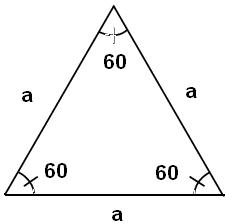Figure 1: Equilateral triangle

2. Isosceles Triangle:

An Isosceles triangle has two congruent sides and the angles opposite the congruent sides are equal in size.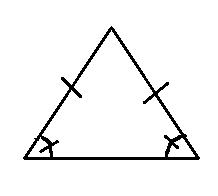Figure 2: Isosceles Triangle

3. Scalene Triangle:

All the three sides and angles of Scalene triangles are different.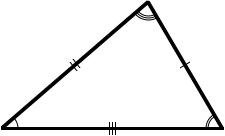Figure 3: Scalene Triangle

4. Right Triangle:

A Right triangle is one in which one angle is 900 and the other two angles are complementary (sum of two angles equal to 900). If two sides of the triangle are known, length of the third side is calculated by the “Pythagorean Theorem” which states that if the length of the sides forming the right angle are given as ‘a’ and ‘b’ and the side opposite to the right angle is called hypotenuse and is given as ‘c’, then c2=a2+b2Figure 4: Right angled triangle

4.1 Right triangle where the angles are 30°, 60°, and 90°:

This is one of the ‘standard’ triangles; the sides are always in the ratio 1:√3:2

Notice that the shortestest side (1) is opposite the smallest angle (30°), and the longest side (2) is opposite the largest angle (90°)Figure 5: Right triangle with 30°, 60°, and 90°

4.2 Right triangle where the angles are 45°, 45°, and 90°:

This is one of the ‘standard’ triangles; the sides are always in the ratio 1:1:√2, where √2 is the hypotenuse (longest side). This can be derived from Pythagoras’ Theorem. Because the base angles are the same (both 45°) the two legs are equal and so the triangle is also isosceles.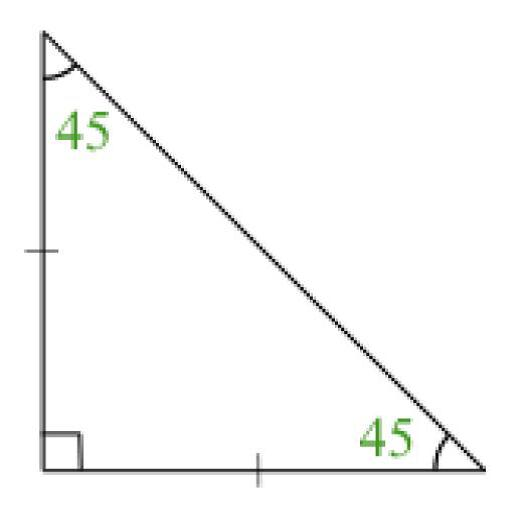Figure 5: Right triangle with 45°, 45°, and 90°

5. Obtuse Triangle:

One of the angles of this triangle is greater than 90° but less than 1800; only one angle can be an obtuse angle and it is impossible to have an equilateral obtuse triangle.

The Figure below shows an example of obtuse angled triangle in which angle is 90° < B <1800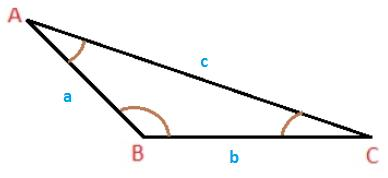Figure 6: Obtuse angled triangle

6. Acute angle:

All the three angles of triangles are greater than 00 but less than 90°.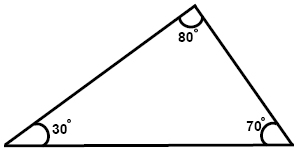Figure 7: Obtuse angled triangle

1. Sum of the interior angles of a triangle is equal to 1800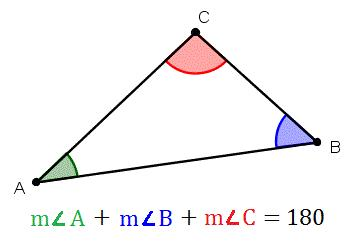2. Exterior angle of a triangle is equal to the sum of the two opposite interior angles. i.e measure of angle A= measure of angle (C +D)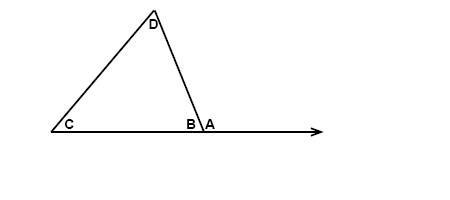3. Length of the third side of a triangle is greater than the difference of other two sides and smaller than the sum of the other two sides. i.e. 305-225 < X < 305+2254. The Side opposite to greatest angle in a triangle is the longest side and the side opposite to the smallest angle is the smallest side.

Related Formulae:

i. Area of any given triangle with base b and height h is given as

Area = bh/2

ii. Area of any triangle with sides a, b, c is given byiii. Perimeter of a triangle when a, b and c are length of sides,

Perimeter = a + b + c

Although most takers of the GRE and GMAT tests do have a basic understanding of these concepts, keeping a ready reckoner of the concepts helps to refresh rules and gear up before the test day. Option Training Institute, Dubai, pays immense attention to inculcating in students a deep and detailed understanding of math concepts, believing that this understanding is what ensures a good score to a large extent. Being skilled with advanced math concepts is impressive but finally do remember that both the GRE and are not advanced math tests. Scoring on these tests depends significantly on having basics clear.